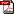アドバンスシミュレーション Vol.26, 西原 慧径, Although Laue-RISM calculates the solute-solvent correlation functions as the same as 3D-RISM, a Laue representation is imposed on the correlation functions, which are periodic on the xy-plane and not periodic along the z-direction. It is possible to apply the Fourier transformation technique on the xy-plane. However, one must deal with the correlation functions in real space along the z-direction. This treatment of the correlation functions allows us to calculate the isolated slab in the solvent system. In addition, we do not need the canonical condition , and the total charge of the solvent system is automatically optimized to screen the electrostatic potential of the solute system. This means that we can obtain the solvent distribution under the grand canonical condition in the solvent system. For example, if the solute slab has a +1.0e charge, the Laue-RISM calculation leads to a solvent distribution whose total charge is -1.0e.(PDF：244kB)

ダウンロードする